# Excel date functions - formula examples of DATE, TODAY, etc.

This is the final part of our Excel Date Tutorial that offers an overview of all Excel date functions, explains their basic uses and provides lots of formula examples.

Microsoft Excel provides a ton of functions to work with dates and times. Each function performs a simple operation and by combining several functions within one formula you can solve more complex and challenging tasks.

In the previous 12 parts of our Excel dates tutorial, we have studied the main Excel date functions in detail. In this final part, we are going to summarize the gained knowledge and provide links to a variety the formula examples to help you find the function best suited for calculating your dates.

## Excel DATE function

`DATE(year, month, day)` returns a serial number of a date based on the year, month and day values that you specify.

When it comes to working with dates in Excel, DATE is the most essential function to understand. The point is that other Excel date functions not always can recognize dates entered in the text format. So, when performing date calculations in Excel, you'd better supply dates using the DATE function to ensure the correct results.

Here are a few Excel DATE formula examples:

`=DATE(2015, 5, 20)` - returns a serial number corresponding to 20-May-2015.

`=DATE(YEAR(TODAY()), MONTH(TODAY()), 1)` - returns the first day of the current year and month.

`=DATE(2015, 5, 20)-5` - subtracts 5 days from May 20, 2015.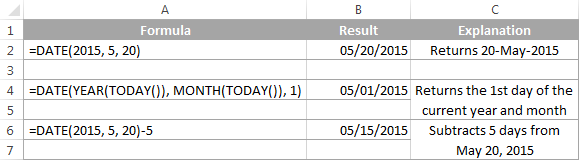At first sight, the Excel DATE function looks very simple, however, it does have a number of specificities pointed out in the Excel DATE tutorial.

Below you will find a few more examples where the Excel DATE function is part of bigger formulas:

## Excel TODAY function

The `TODAY()` function returns today's date, exactly as its name suggests.

TODAY is arguably one of the easiest Excel functions to use because it has no arguments at all. Whenever you need to get today's date in Excel, enter the following formula is a cell:

`=TODAY()`

Apart from this obvious use, the Excel TODAY function can be part of more complex formulas and calculations based on today's date. For example, to add 7 days to the current date, enter the following formula in a cell:

`=TODAY()+7`

To add 30 weekdays to today's date excluding weekend days, use this one:

`=WORKDAY(TODAY(), 30)`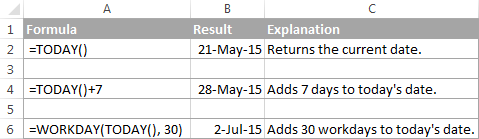Note. The date returned by the TODAY function in Excel updates automatically when your worksheet is recalculated to reflect the current date.

For more formula examples demonstrating the use of the TODAY function in Excel, please check out the following tutorials:

## Excel NOW function

`NOW()` function returns the current date and time. As well as TODAY, it does not have any arguments. If you wish to display today's date and current time in your worksheet, simply put the following formula in a cell:

`=NOW()`

Note. As well as TODAY, Excel NOW is a volatile function that refreshes the returned value every time the worksheet is recalculated. Please note, the cell with the NOW() formula does not auto update in real-time, only when the workbook is reopened or the worksheet is recalculated.

To force the spreadsheet to recalculate, and consequently get your NOW formula to update its value, press either Shift+F9 to recalculate only the active worksheet or F9 to recalculate all open workbooks.

To make the NOW() function automatically update every second or so, a VBA macro is needed (a few examples are available here).

## Excel DATEVALUE function

`DATEVALUE(date_text)` converts a date in the text format to a serial number that represents a date.

The DATEVALUE function understands plenty of date formats as well as references to cells that contain "text dates". DATEVALUE comes in really handy to calculate, filter or sort dates stored as text and convert such "text dates" to the Date format.

A few simple DATEVALUE formula examples follow below:

`=DATEVALUE("20-may-2015")`

`=DATEVALUE("5/20/2015")`

`=DATEVALUE("may 20, 2015")`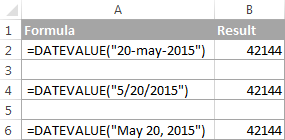And the following examples demonstrate how the DATEVALUE function can help with solving real-life tasks:

## Excel TEXT function

In the pure sense, the TEXT function cannot be classified as one of Excel date functions because it can convert any numeric value, not only dates, to a text string.

With the TEXT(value, format_text) function, you can change the dates to text strings in a variety of formats, as demonstrated in the following screenshot.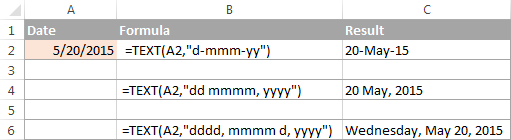Note. Though the values returned by the TEXT function may look like usual Excel dates, they are text values in nature and therefore cannot be used in other formulas and calculations.

Here are a few more TEXT formula examples that you may find helpful:

## Excel DAY function

`DAY(serial_number)` function returns a day of the month as an integer from 1 to 31.

Serial_number is the date corresponding to the day you are trying to get. It can be a cell reference, a date entered by using the DATE function, or returned by other formulas.

Here are a few formula examples:

`=DAY(A2)` - returns the day of the date in A2

`=DAY(DATE(2015,1,1))` - returns the day of 1-Jan-2015

`=DAY(TODAY())` - returns the day of today's date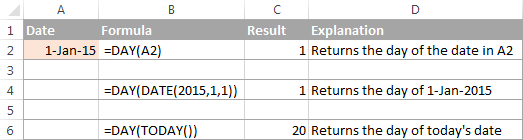You can find more DAY formula examples by clicking the following links:

## Excel MONTH function

`MONTH(serial_number)` function in Excel returns the month of a specified date as an integer ranging from 1 (January) to 12 (December).

For example:

`=MONTH(A2)` - returns the month of a date in cell A2.

`=MONTH(TODAY())` - returns the current month.

The MONTH function is rarely used in Excel date formulas on its own. Most often you would utilize it in conjunction with other functions as demonstrated in the following examples:

For the detail explanation of the MONTH function's syntax and plenty more formula examples, please check out the following tutorial: Using the MONTH function in Excel.

## Excel YEAR function

`YEAR(serial_number)` returns a year corresponding to a given date, as a number from 1900 to 9999.

The Excel YEAR function is very straightforward and you will hardly run into any difficulties when using it in your date calculations:

`=YEAR(A2)` - returns the year of a date in cell A2.

`=YEAR("20-May-2015")` - returns the year of the specified date.

`=YEAR(DATE(2015,5,20))` - a more reliable method to get the year of a given date.

`=YEAR(TODAY())` - returns the current year.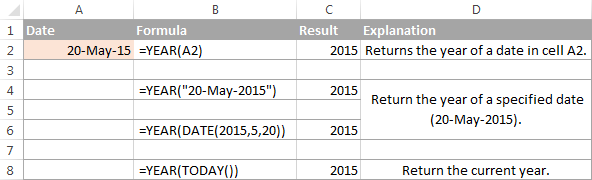## Excel EOMONTH function

`EOMONTH(start_date, months)` function returns the last day of the month a given number of months from the start date.

Like most of Excel date functions, EOMONTH can operate on dates input as cell references, entered by using the DATE function, or results of other formulas.

• A positive value in the `months` argument adds the corresponding number of months to the start date, for example:

`=EOMONTH(A2, 3)` - returns the last day of the month, 3 months after the date in cell A2.

• A negative value in the `months` argument subtracts the corresponding number of months from the start date:

`=EOMONTH(A2, -3)` - returns the last day of the month, 3 months before the date in cell A2.

• A zero in the `months` argument forces the EOMONTH function to return the last day of the start date's month:

`=EOMONTH(DATE(2015,4,15), 0)` - returns the last day in April, 2015.

• To get the last day of the current month, enter the TODAY function in the `start_date` argument and 0 in `months`:

`=EOMONTH(TODAY(), 0)`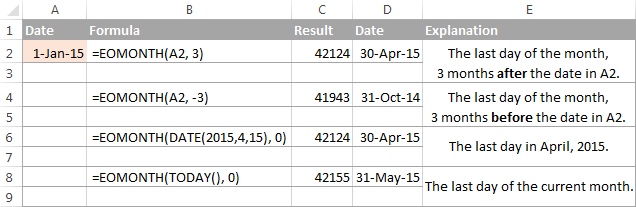You can find a few more EOMONTH formula examples in the following articles:

## Excel WEEKDAY function

`WEEKDAY(serial_number,[return_type])` function returns the day of the week corresponding to a date, as a number from 1 (Sunday) to 7 (Saturday).

• Serial_number can be a date, a reference to a cell containing a date, or a date returned by some other Excel function.
• Return_type (optional) - is a number that determines which day of the week shall be considered the first day.

You can find the complete list of available return types in the following tutorial: Calculating days of week in Excel (WEEKDAY function).

And here are a few WEEKEND formula examples:

`=WEEKDAY(A2)` - returns the day of the week corresponding to a date in cell A2; the 1st day of the week is Sunday (default).

`=WEEKDAY(A2, 2)` - returns the day of the week corresponding to a date in cell A2; the week begins on Monday.

`=WEEKDAY(TODAY())` - returns a number corresponding to today's day of the week; the week begins on Sunday.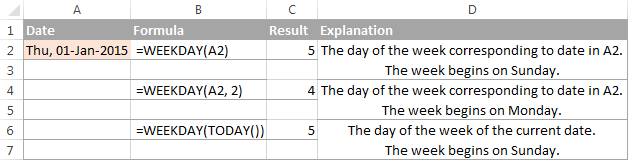The WEEKDAY function can help you determine which dates in your Excel sheet are working days and which ones are weekend days, and also sort, filter or highlight workdays and weekends:

## Excel DATEDIF function

`DATEDIF(start_date, end_date, unit)` function is specially designed to calculate the difference between two dates in days, months or years.

Which time interval to use for calculating the date difference depends on the letter you enter in the last argument:

`=DATEDIF(A2, TODAY(), "d")` - calculates the number of days between the date in A2 and today's date.

`=DATEDIF(A2, A5, "m")` - returns the number of complete months between the dates in A2 and B2.

`=DATEDIF(A2, A5, "y")` - returns the number of complete years between the dates in A2 and B2.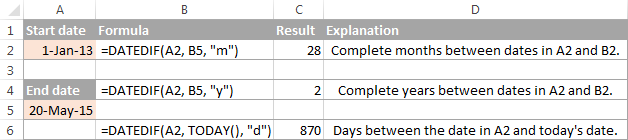These are just the basic applications of the DATEDIF function and it is capable of much more, as demonstrated in the following examples:

## Excel WEEKNUM function

`WEEKNUM(serial_number, [return_type])` - returns the week number of a specific date as an integer from 1 to 53.

For example, the below formula returns 1 because the week containing January 1 is the first week in the year.

`=WEEKNUM("1-Jan-2015")`

The following tutorial explains all the specificities on the Excel WEEKNUM function: WEEKNUM function - calculating week number in Excel.

Alternatively you can skip directly to one of the formula examples:

## Excel EDATE function

`EDATE(start_date, months)` function returns the serial number of the date that is the specified number of months before or after the start date.

For example:

`=EDATE(A2, 5)` - adds 5 months to the date in cell A2.

`=EDATE(TODAY(), -5)` - subtracts 5 months from today's date.

For a detailed explanation of EDATE formulas illustrated with formula examples, please see: Add or subtract months to a date with EDATE function.

## Excel YEARFRAC function

`YEARFRAC(start_date, end_date, [basis])` function calculates the proportion of the year between 2 dates.

This very specific function can be used to solve practical tasks such as calculating age from date of birth.

## Excel WORKDAY function

`WORKDAY(start_date, days, [holidays])` function returns a date N workdays before or after the start date. It automatically excludes weekend days from calculations as well as any holidays that you specify.

This function is very helpful for calculating milestones and other important events based on the standard working calendar.

For example, the following formula adds 45 weekdays to the start date in cell A2, ignoring holidays in cells B2:B8:

`=WORKDAY(A2, 45, B2:B85)`

For the detailed explanation of WORKDAY's syntax and more formula examples, please check out WORKDAY function - add or subtract workdays in Excel.

## Excel WORKDAY.INTL function

`WORKDAY.INTL(start_date, days, [weekend], [holidays])` is a more powerful variation of the WORKDAY function introduced in Excel 2010 and also available Excel 2013 and 2016.

WORKDAY.INTL allows calculating a date N number of workdays in the future or in the past with custom weekend parameters.

For example, to get a date 20 workdays after the start date in cell A2, with Monday and Sunday counted as weekend days, you can use either of the following formulas:

`=WORKDAY.INTL(A2, 20, 2, 7)`

or

`=WORKDAY.INTL(A2, 20, "1000001")`

Of course, it might be difficult to grasp the essence from this short explanation, but more formula examples illustrated with screenshots will make things really easy: WORKDAY.INTL - calculating workdays with custom weekends.

## Excel NETWORKDAYS function

`NETWORKDAYS(start_date, end_date, [holidays])` function returns the number of weekdays between two dates that you specify. It automatically excludes weekend days and, optionally, the holidays.

For example, the following formula calculates the number of whole workdays between the start date in A2 and end date in B2, ignoring Saturdays and Sundays and excluding holidays in cells C2:C5:

`=NETWORKDAYS(A2, B2, C2:C5)`

You can find a comprehensive explanation of the NETWORKDAYS function's arguments illustrated with formula examples and screenshots in the following tutorial: NETWORKDAYS function - calculating workdays between two dates.

## Excel NETWORKDAYS.INTL function

`NETWORKDAYS.INTL(start_date, end_date, [weekend], [holidays])` is a more powerful modification of the NETWORKDAYS function available in the modern versions of Excel 2010, Excel 2013 and Excel 2016. It also returns the number of weekdays between two dates, but lets you specify which days should be counted as weekends.

Here is a basic NETWORKDAYS formula:

`=NETWORKDAYS(A2, B2, 2, C2:C5)`

The formula calculates the number of workdays between the date in A2 (start_date) and the date in B2 (end_date), excluding the weekend days Sunday and Monday (number 2 in the weekend parameter), and ignoring holidays in cells C2:C5.

For full details about the NETWORKDAYS.INTL function, please see NETWORKDAYS function - counting workdays with custom weekends.

Hopefully, this 10K foot view on the Excel date functions has helped you gain the general understanding of how date formulas work in Excel. If you want to learn more, I encourage you to check out the formula examples referenced on this page. I thank you for reading and hope to see you again on our blog next week!

### 335 responses to "Excel date functions - formula examples of DATE, TODAY, etc."

1.Deepjyothi0212@gmail.com says:

How to get the number of remaining days for a specific date, eg- if A1=29 I need 2 as a return in B1 if today's date is 27, same way if A1=3 I need 3 as a return in B1 if today is the last day of month.
So please suggest any formula for this, if there is any.

2.Christina says:

I need to make daily sign-in sheets for company visitors. Is there any way to make one sign-in sheet and have the working days populate for the rest of the month?

•Yogesh Abhyankar says:

I can help you out for your query.But tell me one thing that you said "1 sign-in-sheet and have the working days populate for the rest of the month". Does this mean you want to calculate the present days for the visitors or the remaining days of that particular month?

3.khan says:

start date and end date is greater than 6 months then count full year.
for example
01-01-2000 to 02-04-2019 the answer is 28 year 6 months and 1 day
but i get the only 29 year only
01-01-2000 to 01-04-2019 the answer is 28 year 5 months and 30 days
but i get the only 28 year only
any formula in excel

•raju says:

try to this type of formula you will get

•rajuraju@gmail.com says:

try it
=DATEDIF(B1,B2,"y")&" Years " & DATEDIF(B1,B2,"ym")&" months " & DATEDIF(B1,B2,"md")&" days "

4.Tyler Price says:

Hi,

If I use the formula for today's date, will the date update every day?
I'm looking for a formula to log the current date when a certain value is reached, but if the TODAY formula updates to current day I won't be able to log the date the value is reached.
Can someone please clarify how this works? And if it does only give the current date, can you please let me know if there is a formula to log the current date and not update daily?

Thanks,
Tyler

•Rob says:

not sure what you are asking, current date is not current if it doesn't update

•Svetlana Cheusheva (Ablebits.com Team) says:

Hi Tyler,

Yes, the TODAY formula updates automatically to always show the current date.

If you are looking to insert today's date as an unchangeable time stamp, this can be done with the Ctrl + ; shortcut or a more complex formula that uses a circular reference. You can find full details in How to insert today date & current time as unchangeable time stamp. However, using circular references in Excel is always a risk, so please be sure to weigh all pros and cons carefully before using that formula in your worksheets.

•IJAZ AHMAD says:

=IF(B9>0, TODAY(), "" )

8 | A | B |
9 | 12/14/2019 | Reachable value |
10 | | If Reachable is Null then A-10 show is empty |

5.Severin says:

I need to calculate prorated days for real estate closings automatically for the tax prorations. I have everything figured out except I have to manually enter the prorated date. For example, house closes june 1st, it will always calculate days until june 30th. I simply have a formula subtracting june 30th from june 1st to give me number of days, however, i have to constantly monitor the 6/30 date to make sure the year is the following june 30th, I'd like to automate this. how do i enter a formula that says I want this cell to say 6/30/(after todays date)? so, if today is 8/23/19 I want the prorated date to read 6/30/2020. If it were say, 4/30/19, I want the prorated date to read 6/30/19, so always the june 30th after whatever date.

6.abdul rahman says:

i try to find the remaining day, i try all formula but showing only "VALUE" command only
what i want to do..?

7.Avi Kenaani says:

I have a field like "Thursday, 11/7/2019" how to extract only the date without the day of the week.

Thank you,

8.Leah Shaull says:

I am trying to find the baseline percentage of training hours that an employe should be at on the current day. So if an employee has 3000 minutes worth of training to do I would like to have a cell that tells me the percentage that the should have completed on that day.

9.Matthew Yorke says:

Hi,

How do I add a leap year into an excel formula. I have one set for the Julian calendar which works off a number per day of the year for each of the 365 days. However, I cannot get it to figure out leap years. The formula I am using at the minute is: =IF(C2="","",DATE(YEAR(TODAY()),1,C5)). C2 is where we put the code and C5 is the date.

Thanks

•Mary Trifuntova (Ablebits.com Team) says:

Hi Matthew,
The same formula you sent will work in leap year too. It will simply consider February 29th as the 60th day of the year.

If however, you need to check if the year is leap or not, here is the formula for you:
=IF(MOD(YEAR(A1), 4), "normal year", "leap year")

Where A1 is the cell with a date.

10.darjan says:

Hi all,
I need formula that will count only number of days that have passed - 1 day, for each month.
=DATEDIF(A2, TODAY(), "d") this formula counts number of days that have passed since specific date, I need end that also.

Which means the final number for January should be 31, for February 28(29), for March 31.

Thank you

11.Mustakeem Qureshi says:

I want list of formulas to change the date range 01/01/2020 - 01/31/2020 with formula.

If in between I 'm changing the date then it should continue from the date i changed.

Thanks,
Mustakeem Qureshi

12.JP says:

Is there a formula or an option that will restrict Now() and Today() function to update automatically? I want them to stay fixed from the day I select "yes" on the cell.

This are my current function commands:
=IF(I4="","",IF(I4="yes",TODAY(),"Check SP"))
=IF(I5="","",IF(I5="yes",NOW(),"Check SP"))

13.JA says:

I'm trying to concatenate 2 dates (arrival and departure) so that the result looks like this: Feb 2 - Feb 5 or, if there's a month boundary: OCT 28 - NOV 20

I can't get a formula to work using DATE or TEXT, etc. For example:
=IF(TEXT(D2,"mmm")),TEXT(E2,"mmm"))),CONCAT(CONCAT(TEXT(D2,"mmm","/",TEXT(D2,"dd"))....

There are no helpful error messages.
Any ideas?

Thanks.

14.Larry Assinewe says:

Could I use =DATE ( for copying another date?

15.ANNA CUELLAR says:

WHAT IS THE FORMULA TO HAVE A DATE CHANGE COLOR (YELLOW) 30 DAYS PRIOR TO THE DATE SHOWN AND CHANGE COLOR (RED) AFTER THE DATE SHOWN

16.Emmanuel says:

Hi,
How do I calculate the days completed based on the ()Todays (current date) from a start date and an end date, please?
Example:
Start Date: 20/04/2020
End Date: 10/05/2020
Today's Date: 26/04/2020
Numbers of days completed:?
What's the formula to calculate the number of days completed, taking into account the end date?

•Alexander Trifuntov (Ablebits.com Team) says:

Hello Emmanuel!

=DATEDIF(A1,TODAY(),"d")

where A1 - Start date.
Hope you’ll find this information helpful.

17.Bonnie Schube says:

What is the formula to put two strings together. I need =DATEDIF(A1,A2,"M") but if the A2 is blank calculate by "today" =DATEDIF(A1,"TODAY(),"M")

•Alexander Trifuntov (Ablebits.com Team) says:

Hello Bonnie!
The formula below will do the trick for you:

=IF(A2="",DATEDIF(A1,TODAY(),"m"), DATEDIF(A1,A2,"m"))

18.Myra says:

Hello,
How do I calculate the collection date when 57 days credit term starts from the end of each transaction week?
Invoice date is April 1, 2020

•Alexander Trifuntov (Ablebits.com Team) says:

Hello Myra!
For me to be able to help you better, please describe your task in more detail. Please let me know in more detail what you were trying to find, what formula you used and what problem or error occurred. It’ll help me understand it better and find a solution for you. Thank you.

•Myra says:

Hello Alex,
Sorry for the confusion. I'm not familiar with formulas but I can provide you the details. I'm trying to compute the due date of my invoice. Credit term is 57 days. It starts from the end of transaction week. Invoice date is April 1, 2020. Hope you could help me. Thanks.

•Alexander Trifuntov (Ablebits.com Team) says:

Hello Myra!
If I understand your task correctly, the following formula should work for you:

=B13+(7-WEEKDAY(B1,2))+58

In this formula, the countdown starts on Monday of next week.
I hope this will help, otherwise please do not hesitate to contact me anytime.

•Myra says:

Hello Alex,
Thank you for providing me the formula. May I know how did you come up with this? B1 is for the cell for invoice date? Why using B13, (B1, 2), 58? Sorry not familiar with formulas.
Thank you and stay safe always.

•Alexander Trifuntov (Ablebits.com Team) says:

Hello Myra!
I'm sorry, accidentally wrote an extra digit. Formula -
= B1 + (7-WEEKDAY (B1,2)) + 58
B1 is the invoice date.

•Myra says:

Hello Alex,
What date should I get if invoice is for April 1, 2020 and April 14?
Do I need to change the formula for April 14?
Thanks.

•Alexander Trifuntov (Ablebits.com Team) says:

Hello Myra!
Write this date in B1, and write the formula in any other cell. The formula determines the date of next Monday and adds 57 days

19.Myra says:

Hello Alex,
What if instead of invoice date, it should start from the end of transaction week? Every Friday is the starting date but the invoice date is any date like April 1 Wednesday and 13, 2020 Monday. Thanks

•Alexander Trifuntov (Ablebits.com Team) says:

Hello Myra!
It is a pity that you did not immediately indicate all the conditions. It would take significantly less time.

=A3+(5 > WEEKDAY(A3,2))*(5-WEEKDAY(A3,2))+(5 < WEEKDAY(A3,2))*(12-WEEKDAY(A3,2))+57

A3 is the invoice date.

•Myra says:

Hello Alex,
Sorry for the late reply. Wow, that's a long formula. Will try to understand this. Thank you for helping me. Stay safe always. :)

20.Liz Stewart says:

I can't seem to figure out which combination of formulas i need to create the following result. One column has the date of last purchase of an item and the cell next to it would have a formula that would take that date and add a quantity of days to get the date in the future, and be able to change when the date of last purchase changes. So as a specific example last purchase date is 6/19/2020 the cell next to it then would populate the date 90 days in the future which would be 9/17/2020. Then on 9/17/2020, the cell would update with the date 90 days from that once i enter it in the 'last purchase' cell. Thanks for the help!

21.Baskar Veeraswamy says:

Hi,
Is there any formula to return previous day if the time is between 00:00 ~ 05:00 hrs

•Alexander Trifuntov (Ablebits.com Team) says:

Hello!
If I understand your task correctly, the following formula should work for you:

=IF(AND(HOUR(B1)>0,HOUR(B1)<5),B1-1,B1)

22.Sam says:

Hi,
Am still using excel 2007. So Daily making invoices by date by date. Suddenly when I open the old invoice for checks it’s showing as TODAYs date(current) .. so I need to solve that when opening old document. is there anyone can help me plz

23.Scott D Keller says:

I'm trying to populate todays date when a cell is not blank. Here is what I have for a formula:
=IF(ISBLANK([@[Shipping Release]]),"",TODAY())
I dont want the entire column to change to todays date but instead populate the day that information was input and not automatically update. Any ideas?

24.MD AMJAD says:

Dear Sir,
Please guide me how to change next date into last date in excel

thanks & regard

25.Daniel says:

Hi Expert,

How do I make the cell auto change for Due date (a fixed date) when the date is change (Actual Start Date - Plan Start Date(a fix date)). Can someone help please ?
Example:
Plan Start Date: 01/11/2020 (fixed)
Actual Start Date: 05/11/2020
Difference: 5 days
Due date : 30/10/2020 (fixed) + 5 days - this cell will auto change to 05/11/2020

So basically whenever have changes to Actual Start Date, Due date cell will change automatically based on the difference days count.
What's the formula to use in this situation ?i Thanks !

•Alexander Trifuntov (Ablebits.com Team) says:

Hello!
If I understand your task correctly, the following formula should work for you:

=C1+(B1-A1)

A1 -start date
B1 - actual start date
C1 - due date

•Daniel says:

Hi Alexander,

Thanks for the reply, appreciate it. But that is not that what i want.

Plan Date Actual Start Date
01-Nov (A1) 05-Nov (B1)

Due date
30-Oct (B4)
03-Nov (B5)

My task is to make B4 and B5 to change automatically based on the difference between B1-A1. So my question is, what formula to put in cell B4 and B5 (already has a date in the cell) to make it both auto change based on the day difference. Hope it more clearer for you.

•Alexander Trifuntov (Ablebits.com Team) says:

Hello!
On our forum, we have already written many times that if there is some value in a cell, then the formula cannot be written into it. Your task can be solved using the VBA macro. It is impossible to solve it using an Excel formula.

26.Arun Kumar Uppara says:

Hi Team,

I have a date in one column A and the severity Level in Column B and i am trying to get the future due date in the column C. If the severity Level is Critical in column C then the future date should be after 30 days from the date mentioned in Column A.

Can anyone help me with this formula.

•Alexander Trifuntov (Ablebits.com Team) says:

Hello!
Sorry, I do not fully understand the task. What does "severity Level"? Please describe your problem in more detail. Include an example of the source data and the result you want to get. It’ll help me understand your request better and find a solution for you.

•Arun Kumar Uppara says:

Hi Team,

I am Cyber security Analyst and i am planning to automate teh report which in MS excel.
In one column there will be CVE ID and in next column it's severity level (critical, High, Medium and low) and CVE released date, based on these 3 things i have to update the future date when the Bug or patch has to fixed in our environment.
If the severity Level is Critical then the future date should be after 30 days from the date of CVE release.

•Alexander Trifuntov (Ablebits.com Team) says:

Hello!
If I understand your task correctly, the following formula should work for you:

=IF(B2="critical",C2+30,"")

27.Ade says:

Hi Team,

I was wondering if you could help with task have in hand. So i have a team monthly rota. The dates usually start for example 16th Nov - 13th Dec and another 14th Dec - 10 Dec and so on. In the rota column the dates are format horizontally as below:
16 17 18 19 20 21 22 23
Mon Tue Wed Thur Fri Sat Sun Mon. and so on.

Task: Am trying to populate data from the rota and another worksheet dynamically, have actually complete the task. But, need to select each month of the from a drop down menu and the data for that month will be populate along with the already VLOOPUP formulars.

Problem: How do i convert does weekly dates to 1 months and create a drop down menu as per the rota 16th Nov - 13th Dec and 14th Dec - 10 Dec list.

So i understand in formula is: =IF(above weekly date = 16th Nov - 13th Dec from dropdowm list, VLOOPUP(A1, ARRAY, COLUMN, FALSE), "","")

I can as well have a separate sheet where i can store the weekly date in month and create a validation list from there.

I hope this make sence, if not i can send you my screenshot data and you can have a look.

Looking forward to your swift response.

Thanks

28.Erik says:

Hello,

I have a document that has a date in text form listed like Nov/19. When I use the date value formula, the formula converts it to Nov/20. Is there a formula I can use that will convert the text to the correct date?

•Alexander Trifuntov (Ablebits.com Team) says:

Hello!
Unfortunately, I was unable to repeat your mistake. Please state exactly how your date is written. What formula are you using? What is the default date format?

29.Kam says:

I need to include start date as well end date so what to do. I just did A2-B2+1. any other formula

•Alexander Trifuntov (Ablebits.com Team) says:

Hi,
I’m sorry but your task is not entirely clear to me. Could you please describe it in more detail? What result do you want to get? Give an example of the source data and the expected result.

30.Andrew Bedford says:

I’m a senior nurse trying to figure out what formula I can use to get the duration (in hours) between 2 dates with times.
The way the cells are configured is
08/10/2018 06:00
06/11/2018 19:00

•Alexander Trifuntov (Ablebits.com Team) says:

Hello!
Use a subtraction formula

=B2-A2

In a cell with a formula, apply a custom time format

"37:30:55"

I hope this will help, otherwise please do not hesitate to contact me anytime.

•Andrew bedford says:

Amazing thank you

31.Aimee says:

Is there a way to have the current date that will not update? I'm trying to track compliance dates - so for example when column D=0 column E will show the date that D first equaled 0.

•Alexander Trifuntov (Ablebits.com Team) says:

Hello!
The date in the cell will not change only if it is entered as a timestamp.
To write in cell E1 the date when the value was entered in D1, you need to use a VBA macro.

•aimee says:

Yes! - Thank you!!

32.Ade says:

Hi Team. I have calendar month, range from 1 to 30 day in cell A1 to A30 to and i have in cell A10=14, A11= 7. I have another work sheet call STAT and i wanted to display the SUM of A10 and A11 to dynamically for each day. The issue for me is looping each day dynamically. Hope you can help

•Alexander Trifuntov (Ablebits.com Team) says:

Hi,
I’m sorry but your task is not entirely clear to me. For me to be able to help you better, please describe your task in more detail. Please specify what you were trying to find, what formula you used and what problem or error occurred. Give an example of the source data and the expected result.
It’ll help me understand it better and find a solution for you.

33.Robert Harrison says:

I have a monthly payments due date what is the formula? Also I have couple of yearly (annually) payment due date so what is the formula for yearly date?

•Alexander Trifuntov (Ablebits.com Team) says:

Hello!